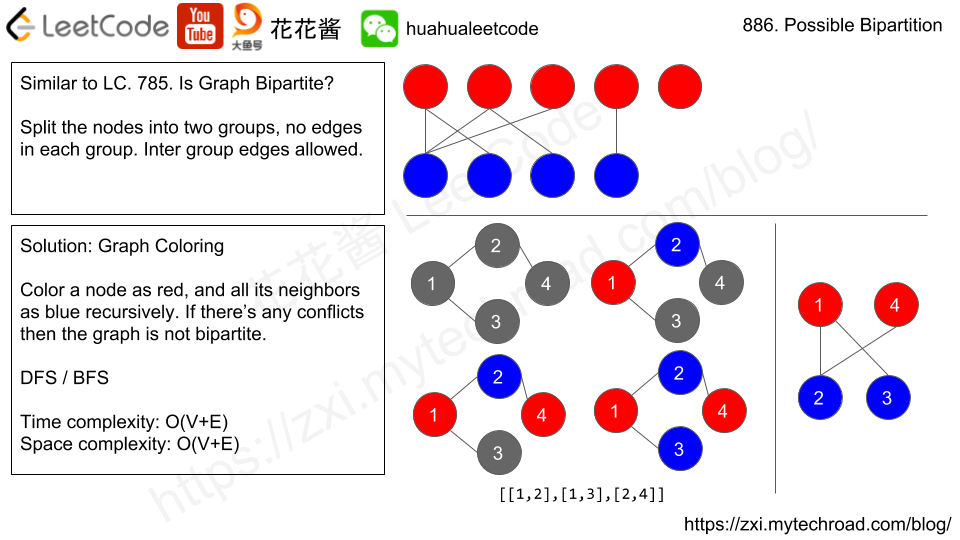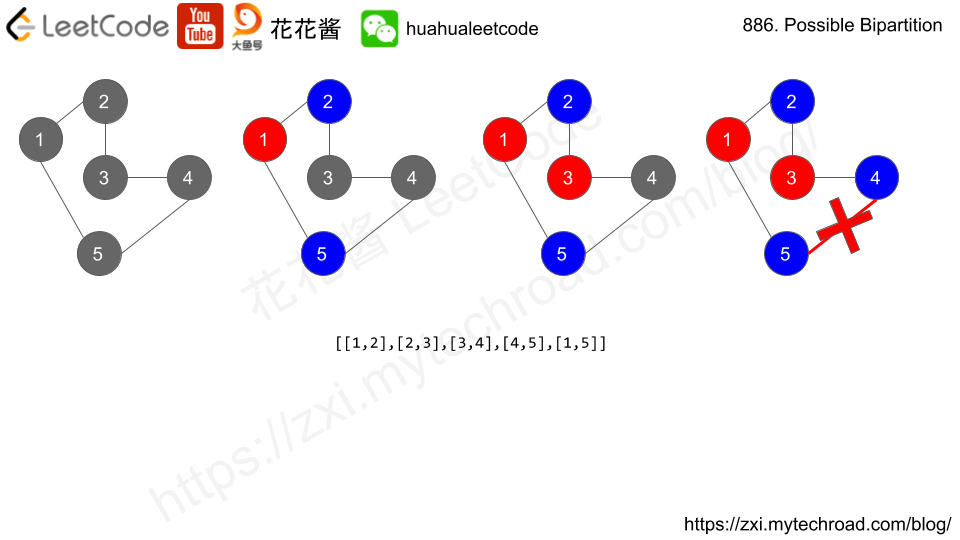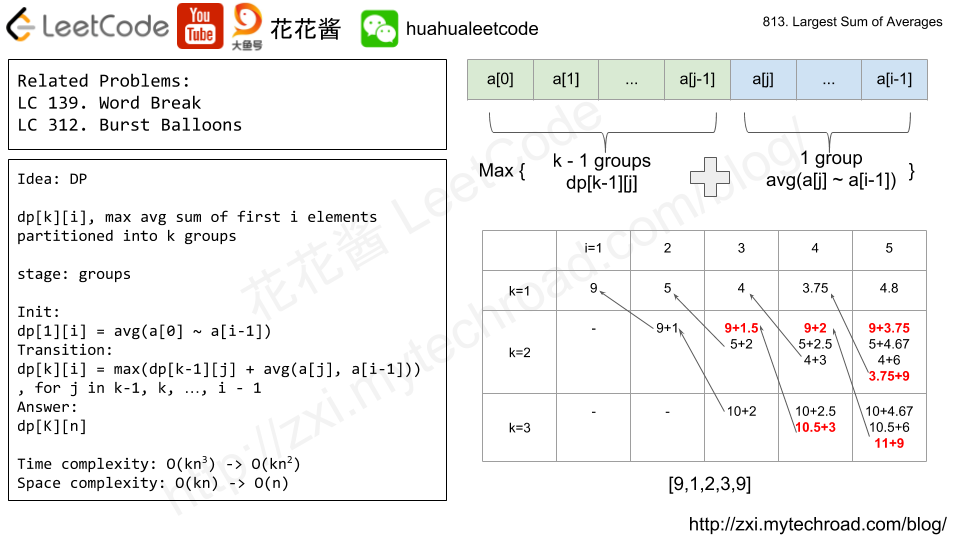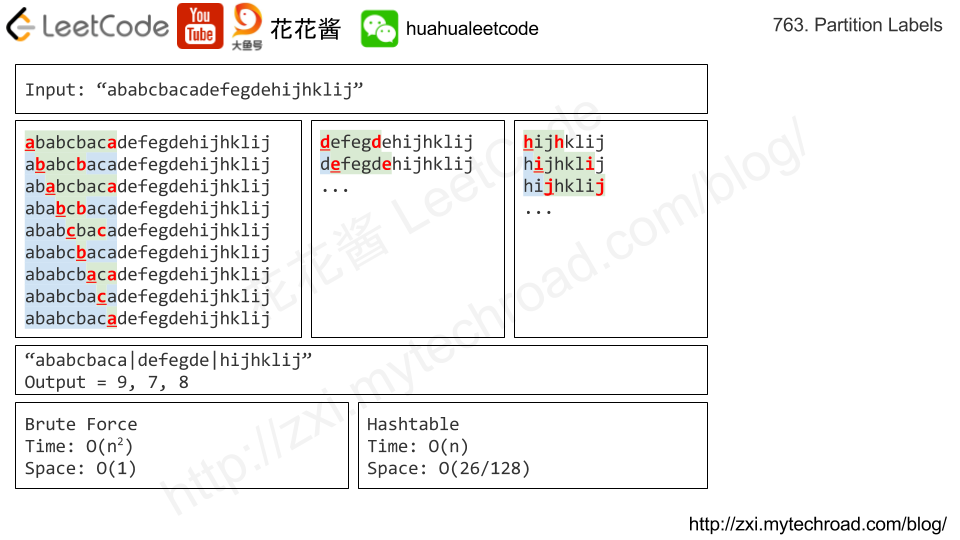# Problem

https://leetcode.com/problems/reorder-log-files/description/

You have an array of logs.  Each log is a space delimited string of words.

For each log, the first word in each log is an alphanumeric identifier.  Then, either:

• Each word after the identifier will consist only of lowercase letters, or;
• Each word after the identifier will consist only of digits.

We will call these two varieties of logs letter-logs and digit-logs.  It is guaranteed that each log has at least one word after its identifier.

Reorder the logs so that all of the letter-logs come before any digit-log.  The letter-logs are ordered lexicographically ignoring identifier, with the identifier used in case of ties.  The digit-logs should be put in their original order.

Return the final order of the logs.

Example 1:

Input: ["a1 9 2 3 1","g1 act car","zo4 4 7","ab1 off key dog","a8 act zoo"]
Output: ["g1 act car","a8 act zoo","ab1 off key dog","a1 9 2 3 1","zo4 4 7"]


Note:

1. 0 <= logs.length <= 100
2. 3 <= logs[i].length <= 100
3. logs[i] is guaranteed to have an identifier, and a word after the identifier.

# Solution: Partition + Sort

1. partition the array such that all digit logs are after all letter logs
2. sort the letter logs part based on the log content

Time complexity: O(n + aloga)

Space complexity: O(n)

# Problem

Given an array of 2n integers, your task is to group these integers into n pairs of integer, say (a1, b1), (a2, b2), …, (an, bn) which makes sum of min(ai, bi) for all i from 1 to n as large as possible.

Example 1:

Input: [1,4,3,2]

Output: 4
Explanation: n is 2, and the maximum sum of pairs is 4 = min(1, 2) + min(3, 4).


Note:

1. n is a positive integer, which is in the range of [1, 10000].
2. All the integers in the array will be in the range of [-10000, 10000].

# Solution 1: Sorting

Time complexity: O(nlogn)

Space complexity: O(1)

# Solution 2: HashTable

Time complexity: O(n + max(nums) – min(nums))

Space complexity: O(max(nums) – min(nums))

# Problem

Given a set of N people (numbered 1, 2, ..., N), we would like to split everyone into two groups of any size.

Each person may dislike some other people, and they should not go into the same group.

Formally, if dislikes[i] = [a, b], it means it is not allowed to put the people numbered a and b into the same group.

Return true if and only if it is possible to split everyone into two groups in this way.

Example 1:

Input: N = 4, dislikes = [[1,2],[1,3],[2,4]]
Output: true
Explanation: group1 [1,4], group2 [2,3]


Example 2:

Input: N = 3, dislikes = [[1,2],[1,3],[2,3]]
Output: false


Example 3:

Input: N = 5, dislikes = [[1,2],[2,3],[3,4],[4,5],[1,5]]
Output: false


Note:

1. 1 <= N <= 2000
2. 0 <= dislikes.length <= 10000
3. 1 <= dislikes[i][j] <= N
4. dislikes[i] < dislikes[i]
5. There does not exist i != j for which dislikes[i] == dislikes[j].# Solution: Graph Coloring

Color a node with one color, and color all it’s disliked nodes with another color, if can not finish return false.

Time complexity: O(V+E)

Space complexity: O(V+E)

# Problem

https://leetcode.com/problems/largest-sum-of-averages/description/

We partition a row of numbers A into at most K adjacent (non-empty) groups, then our score is the sum of the average of each group. What is the largest score we can achieve?

Note that our partition must use every number in A, and that scores are not necessarily integers.

Example:
Input:
A = [9,1,2,3,9]
K = 3
Output: 20
Explanation:
The best choice is to partition A into , [1, 2, 3], . The answer is 9 + (1 + 2 + 3) / 3 + 9 = 20.
We could have also partitioned A into [9, 1], , [3, 9], for example.
That partition would lead to a score of 5 + 2 + 6 = 13, which is worse.


Note:

• 1 <= A.length <= 100.
• 1 <= A[i] <= 10000.
• 1 <= K <= A.length.
• Answers within 10^-6 of the correct answer will be accepted as correct.

# IdeaDP, use dp[k][i] to denote the largest average sum of partitioning first i elements into k groups.

### Init

dp[i] = sum(a ~ a[i – 1]) / i, for i in 1, 2, … , n.

### Transition

dp[k][i] = max(dp[k – 1][j] + sum(a[j] ~ a[i – 1]) / (i – j)) for j in k – 1,…,i-1.

that is find the best j such that maximize dp[k][i]

largest sum of partitioning first j elements (a ~ a[j – 1]) into k – 1 groups (already computed)

+ average of a[j] ~ a[i – 1] (partition a[j] ~ a[i – 1] into 1 group).

dp[K][n]

# Solution 1: DP

Time complexity: O(kn^2)

Space complexity: O(kn)

# Solution 2: DFS + memoriation

## C++

Problem:

A string S of lowercase letters is given. We want to partition this string into as many parts as possible so that each letter appears in at most one part, and return a list of integers representing the size of these parts.

Example 1:Solution 0: Brute Force

Time complexity: O(n^2)

Space complexity: O(1)

C++

Python

Solution 1: Greedy

Time complexity: O(n)

Space complexity: O(26/128)

C++

Java

Python3

Mission News Theme by Compete Themes.